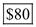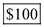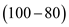## Quiz 7 : Consumers, Producers, and the Efficiency of MarketsLooking for Economics Homework Help?# Quiz 7 : Consumers, Producers, and the Efficiency of Markets

Producer surplus: Producer surplus can be calculated as follows:Thus, producer surplus is. Consumer surplus: Consumer surplus can be calculated as follows:Thus, consumer surplus is. Therefore, the consumer surplus is \$20larger than producer. Hence, option 'a ' is correct.

Consumer surplus is given by the difference between the willingness of the consumer to pay and the actual price he pays. Consumer surplus= Maximum price willing to pay by the buyer - Actual price paid. a) M's consumer surplus= \$80, Actual price paid or market price= 120. Maximum willing price can be calculated by submitting these values in the formula. Consumer surplus= Maximum price willing to pay by the buyer - Actual price paid. \$80 = Maximum price willing to pay by the buyer - \$120 Maximum price willing to pay by the buyer= \$80 +\$ 120 = \$ 200. Maximum price willing to pay by the M is = \$200 b) When actual price paid is \$90. Consumer surplus= Maximum price willing to pay by the buyer - Actual price paid. Actual price paid= \$90 Maximum willing price =\$ 200 Consumer surplus= \$200 -\$90 = \$ 110. Thus, when actual price is \$90, consumer surplus is \$110 c) If the actual market price is \$250, then the consumer surplus is zero because the price of product is greater than the willingness to pay of the consumer. Thus, consumer surplus is zero.

Consumer surplus is equal to the buyers' willingness to pay for a good minus the amount they actually pay for it. It measures the benefit buyers get from participating in a market. Consumer surplus is computed by finding the area below the demand curve and above the price. Thus, consumer surplus is closely related to the demand curve for a product. The demand curve is the graphical illustration of a demand schedule, which is based on the buyers' willingness to pay.